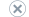# Maximum capacity specifications (Analysis Services)

Applies to:SQL Server Analysis ServicesAzure Analysis ServicesPower BI Premium

The following tables specify the maximum sizes and numbers of various objects defined in SQL Server Analysis Services components under different server deployment modes.

This topic contains the following sections:

Multidimensional and Data Mining (DeploymentMode=0)

SharePoint (DeploymentMode=1)

Tabular (DeploymentMode=2)

## Multidimensional and Data Mining (DeploymentMode=0)

MOLAP storage mode, which stores both data and metadata, has additional physical limits on file sizes. String store files are a maximum size of 4 GB by default. If you require larger files for string stores, you can specify a different string storage architecture. For more information, see Configure String Storage for Dimensions and Partitions.

Object Maximum sizes/numbers
Databases in an instance 2^31-1 = 2,147,483,647
Dimensions in a database 2^31-1 = 2,147,483,647
Attributes in a dimension 2^31-1 = 2,147,483,647
Members in a dimension attribute 2^31-1 = 2,147,483,647
User-defined hierarchies in a dimension 2^31-1 = 2,147,483,647
Levels in a user-defined hierarchy 2^31-1 = 2,147,483,647
Cubes in a database 2^31-1 = 2,147,483,647
Measure groups in a cube 2^31-1 = 2,147,483,647
Measures in a measure group 2^31-1 = 2,147,483,647
Calculations in a cube 2^31-1 = 2,147,483,647
KPIs in a cube 2^31-1 = 2,147,483,647
Actions in a cube 2^31-1 = 2,147,483,647
Partitions in a cube 2^31-1 = 2,147,483,647
Translations in a cube 2^31-1 = 2,147,483,647
Aggregations in a partition 2^31-1 = 2,147,483,647
Cells returned by a query 2^31-1 = 2,147,483,647
Record size of the source query 64K
Length of object names 100 characters
Maximum number of distinct states in a data mining model attribute column 2^31-1 = 2,147,483,647
Maximum number of attributes considered (feature selection) 2^31-1 = 2,147,483,647

For more information about data source limitations for online analytical processing (OLAP) and data mining, see Supported Data Sources (SSAS - Multidimensional), Supported Data Sources (SSAS - Multidimensional), and ASSL Objects and Object Characteristics.

## SharePoint (DeploymentMode=1)

Object Maximum sizes/numbers
Databases in an instance 2^31-1 = 2,147,483,647
Tables in a database 2^31-1 = 2,147,483,647
Columns in a table 2^31-1 = 2,147,483,647

Warning: Total number of columns in a table depends on the total number of Measures and Calculated Columns associated to the same table.

The maximum number of 'Columns + Measures + Calculated Columns' for a table is 2^31-1 = 2,147,483,647
Rows in a table Unlimited

Warning: With the restriction that no single column may contain more than 1,999,999,997 distinct values.
Hierarchies in a table 2^31-1 = 2,147,483,647
Levels in a hierarchy 2^31-1 = 2,147,483,647
Relationships 2^31-1 = 2,147,483,647
Key Columns in a table 2^31-1 = 2,147,483,647
Measures in a table 2^31-1 = 2,147,483,647

Warning: Total number of Measures in a table depends on the total number of Columns and Calculated Columns associated to the same table.

The maximum number of 'Columns + Measures + Calculated Columns' for a table is 2^31-1 = 2,147,483,647
Calculated Columns in a table 2^31-1 = 2,147,483,647

Warning: Total number of Calculated Columns in a table depends on the total number of Columns and Measures associated to the same table.

The maximum number of 'Columns + Measures + Calculated Columns' for a table is 2^31-1 = 2,147,483,647
Cells returned by a query 2^31-1 = 2,147,483,647
Record size of the source query 64K
Length of object names 100 characters

## Tabular (DeploymentMode=2)

The following are theoretical limits. Performance will be diminished at lower numbers.

Object Maximum sizes/numbers
Databases in an instance 16,000
Combined number of tables and columns in a database 16,000
Rows in a table Unlimited

Warning: With the restriction that no single column in the table can have more than 1,999,999,997 distinct values.
Hierarchies in a table 15,999
Levels in a hierarchy 15,999
Relationships 8,000
Key Columns in all table 15,999
Measures in a tables 2^31-1 = 2,147,483,647
Cells returned by a query 2^31-1 = 2,147,483,647
Record size of the source query 64K
Length of object names 512 characters